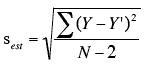### the standard error of estimates (SEE)

The standard error of the estimate (SEE) refers to the deviation of any estimate from the intended values. SEE is a measure of the accuracy of predictions made with a regression line-- in other words, it's about how well a linear model fits the data. The formula for SEE is(Y refers to the dataset and Y' is the mean of the data)

Here's a great video to help students understand the standard error of estimates (SEE).

Another commonly used statistic to measure how well the linear model fits the data is R-squared (the coefficient of determination). And while R^2 is a commonly used statistic, this post offers a more in depth analysis of the good and the bad of R^2. The same author gives you an idea of how to use R^2 in conjunction with SEE and the value of SEE.